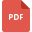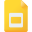# mathematics grade 6 united states tutorialsAddition and Multiplication Properties for Real NumbersFinding Reciprocals of Fractions and Mixed Numbers
Fraction, Grade 6, United States, MathematicsAdding and Subtracting Fractions with Sample QuestionsMultiplying Mixed Numbers Worksheet
Fractions and Multiplication, Grade 6, United States, MathematicsProperties of Triangle: Angle Sum of a Triangle
Properties of Triangle, Grade 6, United States, MathematicsDividing Fractions and Mixed Numbers Worksheet
Fractions and Division, Grade 6, United States, MathematicsEquivalent Fractions by Multiplication and Division
Fraction, Grade 6, United States, MathematicsWorksheet on Equivalent fractions
Fraction, Grade 6, United States, MathematicsMultiplying Fractions by Whole Numbers worksheet
Fraction, Grade 6, United States, MathematicsProbability, Grade 6, United States, MathematicsMultiplying Fractions Worksheet with Solutions
Fractions and Multiplication, Grade 6, United States, MathematicsDividing Fractions and Mixed Numbers
Fractions and Division, Grade 6, United States, MathematicsProbability: Case Study of Rolling a pair of dice
Probability, Grade 6, United States, MathematicsFinding Area of Rectangles and Parallelogram
Parallelogram, Grade 6, United States, Mathematics, Area, GeometryComparing and Ordering Fractions Worksheets
Fraction, Grade 6, United States, MathematicsUsing Oder of Operations Procedure with Fractions
Fraction, Grade 6, United States, MathematicsWorksheet on Order of Operations with Solutions
Fraction, Grade 6, United States, MathematicsRoman Numerals: Counting Methods
Mathematics, Grade 6, United States, Roman NumeralsReducing Fractions to Lowest Equivalent Form
Fraction, Grade 6, United States, MathematicsFinding the Area of a Triangle and Other Composite Shapes
Area, Grade 6, United States, Triangle, Geometry, MathematicsComparing and Ordering Fractions and Mixed Numbers
Fraction, Grade 6, United States, MathematicsExperimental Probability: Theory and Sample Problems
Probability, Grade 6, United States, MathematicsFinding the Volume of Rectangular Prisms
Solid Shapes, Grade 6, United States, Mathematics